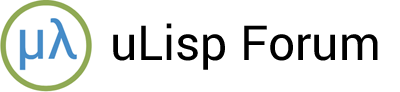# Taking advantage of bit arrays

#1

One of the recent additions to uLisp that may have passed most people by is bit arrays. These were previously part of the array implementation in the ARM, ESP, and RISC-V versions of uLisp, and with Version 4.3 of uLisp are now available in the AVR version of uLisp.

So what are bit arrays, and why would you need them?

Well, variables on microcontrollers often have just two possible values. Examples include digital I/O pins, flags, and true/false logic values. If you’re working with an array of these values it is wasteful of the Lisp workspace to allocate an array of integers; a bit array packs the binary values far more efficiently within the Lisp objects.

### Example

Here’s a simple example of using a bit array, running on an AVR128DA48 board with the AVR Version 4.3 of uLisp.

First we define a two-dimensional 36 x 36 bit array called a:

``````2919> (defvar a (make-array '(36 36) :element-type 'bit :initial-element 0))
a

2824>
``````

We can calculate how many 4-byte Lisp objects this has used from the prompt giving the amount of free memory:

``````2824>  (- 2919 2824)
95
``````

This total of 95 objects is substantially less than if we allocated a Lisp object for every array element, which would be 36 x 36 or 1296 objects.

### Changing and reading a value in a bit array

To change a value in a bit array you use the Lisp setf function, with the aref (array reference) function defining the element you want to change; for example:

``````> (setf (aref a 20 10) 1)
1
``````

This sets the value of the array element (20, 10) to 1. The only allowed values are 0 and 1; any other value will give an error.

To read the value of an element we again use the aref function:

``````> (aref a 20 10)
1
``````

### Printing a two-dimensional bit array

Here’s a simple program to print out a two-dimensional bit array as a matrix of characters, with a “.” representing a zero and a “@” representing a one:

``````(defun bitmap (arr)
"Prints out a two-dimensional bit array as a square of dots and @ signs."
(let* ((dim (array-dimensions arr))
(xd (first dim))
(yd (second dim)))
(dotimes (x xd)
(dotimes (y yd)
(format t " ~a " (if (zerop (aref arr x y)) #\. #\@)))
(terpri))))
``````

### Drawing a spiral

Finally, here’s a program to set a pattern of ones into a two-dimensional bit array:

``````(defun spiral (arr)
"Draws a spiral in a two-dimensional bit array."
(let* ((dim (array-dimensions arr))
(xd (first dim))
(yd (second dim))
(x (truncate xd 2))
(y (truncate yd 2))
(step 2) (xdir -1) (ydir 0) w stop)
(setf (aref arr x y) 1)
(loop
(dotimes (j 2)
(dotimes (i step)
(setf x (+ x xdir) y (+ y ydir))
(when (or (< x 0) (< y 0) (>= x xd) (>= y yd)) (setq stop t) (return))
(setf (aref arr x y) 1))
(when stop (return stop))
(setf w xdir xdir ydir ydir w))
(when stop (return stop))
(incf step 2)
(setf xdir (- xdir) ydir (- ydir)))))
``````

By running (spiral a) followed by (bitmap a) we can see the effect of the spiral program in the Arduino IDE’s Serial Monitor:

#2

I was happy to see them. When you work with such small memory constraints one concern about using a powerful language like Lisp is paying for that expressiveness. I was toying with mine sweeper over the weekend and they were a great way to store a handful of Boolean flags per cell.